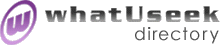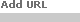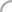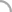SearchThe Directory The Webfor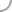CalculusNew! Submit a site

Categories:Online Courses *(7)whatUseek Collection Sites (submit a site ):

Give your site great placement in this category in as little as two business days!

whatUseek Directory Site Listings:

A Physicist's View of Teaching Calculus - Topics in calculus that are important in physics

Are You Ready Review Quizzes - There are review quizzes, on this page, to see, if one is ready to go on and take algebra, calculus or differential equations courses.

Automatic Calculus Solutions - Check calculus homework. Enter a function and click for a step-by-step derivative or integral with each step explained.

Calculus Assistance Egroup Newsgroup - Find help solving calculus problems through a message board, chat, or direct email.

Calculus Made Easier: A Calculus Tutorial - This is an introduction to the basic concepts of calculus. The derivative and integral are explained. Calculus resource links are included.

Calculus Problems and Solutions - Limits and derivatives problems and solutions. A college level tutorial covering differential calculus, along with an introduction to integration.

Calculus Solutions - Solutions to typical calculus problems. Indexed to major texts.

Calculus and Physics Practice Exams - Practice exams for applied calculus and physics in PDF and HTML formats. Detailed solutions to answers are given.

Calculus on the Internet - Tutorials on calculus subjects ranging from precalculus to differential equations. Math tools and resource links.

Calculus on the Web - An internet tutoring utility for learning and practicing calculus. C.O.W. gives the student or interested user the opportunity to learn and practice problems. Instant feedback for the correctness of answers.

CalculusQuest - An online differential calculus textbook including practice activities.

Connected Calculus - This is an applied calculus tutorial. Some prior calculus knowledge might be helpful.

Cyber Calculus - A detailed differential calculus tutorial. Definitions, graphs and precalculus review.

Dan's Math at Home Lessons: Calculus - Features step by step lessons to introductory calculus. Included are function and precalculus preview pages.

Dr. Vogel's Gallery of Calculus Pathologies - Discusses instances of functions that are more difficult to apply calculus formulas to. Examples that are included are discontinuous functions and functions with discontinuous derivatives.

Elliptic Integrals - Asymptotic solutions to symmetric elliptic integrals.

Finite Mathematics and Applied Calculus Resource Page - Features brief tutorials on differential calculus topics. Applied subjects such as maximum and minimum problems and related rates are discussed. Indefinite integrals are introduced as antiderivatives. Includes separate sections on algebra and matrices.

Graphics for the Calculus Classroom - Graphical demonstrations developed by Douglas N. Arnold for the first year calculus student.

Harvey Mudd College Calculus Tutorial - Tutorials covering precalculus, calculus, multivariable calculus, linear algebra and differential equations.

[ 1 2 ]Help build the largest human-edited directory on the web.About   Help   Content Filter   Terms   Privacy Policy© 2018 whatUseek